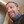# Getting return value From Function with loop

Greetings. This ius my first post. Below is code that calculates the value of a scrabble word.
It works well if I use the println() but cannot get it to return the value in total varable. Thanh you
function score(str)
letterValue = 0
total = 0

dict = Dict(‘a’ => 1, ‘e’ => 1, ‘i’ => 1, ‘o’ => 1, ‘u’ => 1, ‘l’ => 1, ‘n’ => 1,‘r’ => 1,‘s’ => 1,
‘t’ => 1,‘d’ => 2,‘g’ => 2,‘b’ => 3,‘c’ => 3,‘m’ => 3,‘p’ => 3, ‘f’ => 4,‘h’ => 4,‘v’ => 4,‘w’ => 4,
‘y’ => 4,‘k’ => 5, ‘j’ => 8, ‘x’ => 8,‘q’ => 10, ‘z’ => 10)

for character in str
letterValue = dict[character]
total += letterValue
end
end

Using Julia 1.9 and VS Code

``````function score(str)
letterValue = 0
total = 0

dict = Dict('a' => 1, 'e' => 1, 'i' => 1, 'o' => 1, 'u' => 1, 'l' => 1, 'n' => 1, 'r' => 1, 's' => 1,
't' => 1, 'd' => 2, 'g' => 2, 'b' => 3, 'c' => 3, 'm' => 3, 'p' => 3, 'f' => 4, 'h' => 4, 'v' => 4, 'w' => 4,
'y' => 4, 'k' => 5, 'j' => 8, 'x' => 8, 'q' => 10, 'z' => 10)

for character in str
letterValue = dict[character]
total += letterValue
end
total
end
``````

Just add “total” before the last “end” as shown, and it will work.

2 Likes

To be a little more explicit, this is a shortcut for `return total`, which means that when you call the function `result = score(str)`, the `result` variable will contain what you named `total` inside the function

THANK YOU Tried that using score(“apple”) but still no result comes out

tephen1959vsoler

3m

THANK YOU Tried that using score(“apple”) but still no result comes out

Are you sure? Here’s a fresh REPL session:

``````julia> function score(str)
letterValue = 0
total = 0

dict = Dict('a' => 1, 'e' => 1, 'i' => 1, 'o' => 1, 'u' => 1, 'l' => 1, 'n' => 1, 'r' => 1, 's' => 1,
't' => 1, 'd' => 2, 'g' => 2, 'b' => 3, 'c' => 3, 'm' => 3, 'p' => 3, 'f' => 4, 'h' => 4, 'v' => 4, 'w' => 4,
'y' => 4, 'k' => 5, 'j' => 8, 'x' => 8, 'q' => 10, 'z' => 10)

for character in str
letterValue = dict[character]
total += letterValue
end
total
end
score (generic function with 1 method)

julia> score("apple")
9

julia> result = score("apple")
9

julia> result
9
``````
1 Like

Thank you to you all. Got it sorted out with your help

2 Likes